### Prior to SAS Viya

With the creation of SAS Viya, the ability to run DATA Step code in a distributed manner became a reality. Prior to distributed DATA Step, DATA Step programmers never had to think about achieving repeatable results when SAS7BDAT datasets were the sources to their DATA Step code that contains a BY statement. This is because prior to SAS Cloud Analytics Services (CAS), DATA Step ran single-threaded and the source SAS7BDAT dataset was stored on disk. Every time one would run the code we obtained repeatable results because the sequence of rows within the BY group were preserved between runs. To illustrate this, review figures 1, 2, and 3.

Figure 1 is the source SAS7BDAT dataset WORK.TEST1. Notice the sequence of VAR2, especially on row 1 and 4 (i.e., _N_ =1 and 4).

 _n_ VAR1 VAR2 1 1 N 2 1 Y 3 1 Y 4 2 Y 5 2 Y 6 2 N

Figure 1. WORK.TEST1 the original SAS7BDAT dataset

In figure 2, we see a BY statement with variable VAR1. This will ensure VAR1 is in ascending order. We are also using FIRST. processing to identify the first occurrence of the BY group. Because this data is stored on disk and because the DATA Step is executed using a single thread, the result table will be repeatable no matter how many times we run the DATA Step code.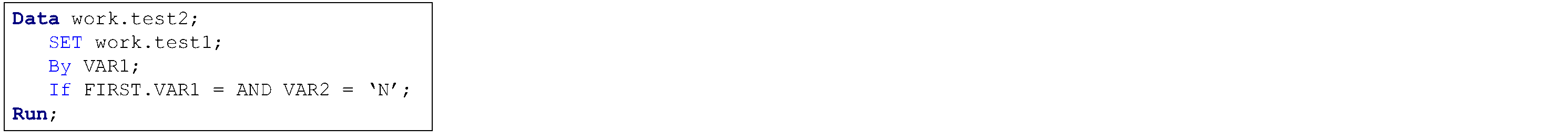Figure 2. Focus on the IF statement, especially VAR2

In figure 3, we see the output SAS7BDAT dataset WORK.TEST2.

 _n_ VAR1 VAR2 1 1 N

Figure 3. WORK.TEST2 result dataset from running the code in Figure 2

In figure 4, we are running the same DATA Step but this time our source and target tables are CAS tables. The source table CASLIB.TEST1 was created by lifting the original SAS7BDAT dataset WORK.TEST1 (review figure 1) into CAS.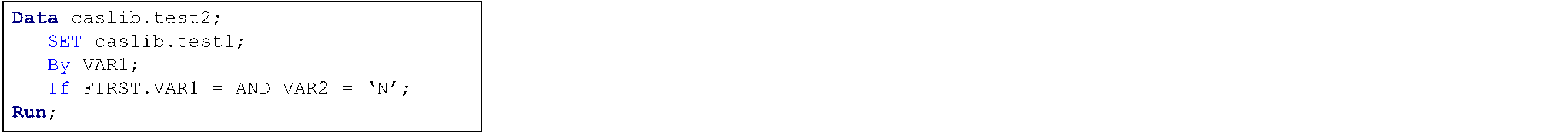Figure 4. DATA Step executing in CAS

In figure 5, we see that the DATA Step logic is being respected in runs 1, 2 and 3; but we are not achieving repeatable results. This is due to CAS running on multiple threads. Note that the BY statement – which will group the data correctly for each BY group – is done on the fly. Also, the BY statement will not preserve the sequence of rows within the BY group between runs.

For some processes, this is not a concern but for others it could be. If you need to obtain repeatable results in DATA Step code that runs distributed in CAS as well as match your SAS 9 single-threaded DATA Step results, I suggest the following workaround be used.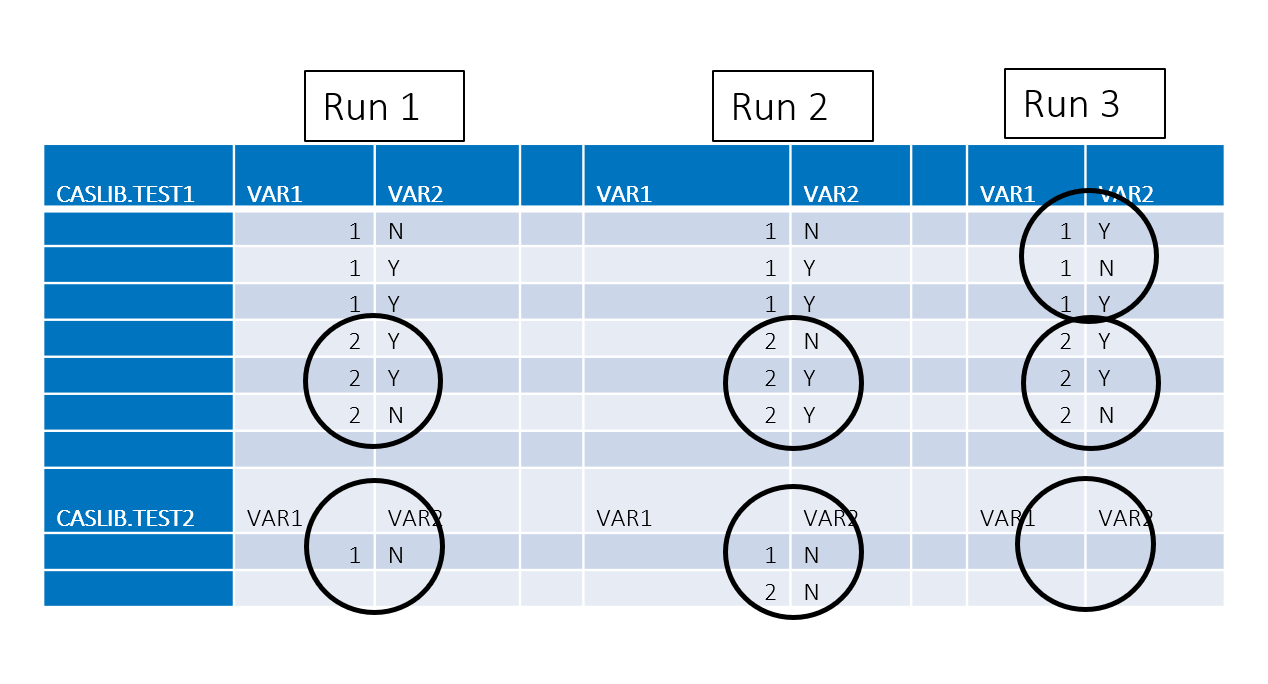Figure 5. DATA Step logic is respected but yields different results with each run

### With SAS Viya

The workaround is very simplistic to understand and implement. For each SAS7BDAT dataset being lifted into a CAS table, see figure 6, we need to add a new variable ROW_ID.

 _n_ VAR1 VAR2 1 1 N 2 1 Y 3 1 Y 4 2 Y 5 2 Y 6 2 N

Figure 6. Original SAS7BDAT dataset source WORK.TEST1

To accomplish this, we will leverage the automatic variable _N_ that is available to all DATA Step programmers. _N_ is initially set to 1. Each time the DATA step loops past the DATA statement, the variable _N_ increments by 1. The value of _N_ represents the number of times the DATA step has iterated. In our case, the value for each row is the row sequence in the original SAS7BDAT dataset. Figure 7 contains the SAS code we ran on the SAS 9.4M5 workspace server or the SAS Viya compute server to add the new variable ROW_ID.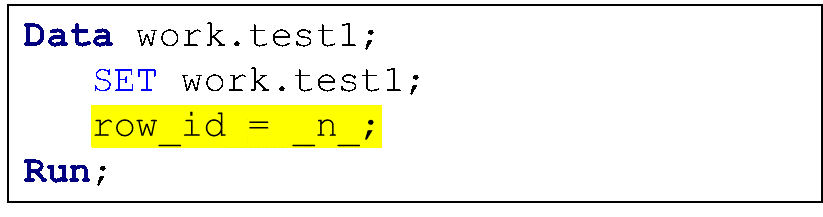Figure 7. Creating the new variable ROW_ID

By reviewing figure 8 we can see the new variable ROW_ID in the SAS7BDAT dataset WORK.TEST1. Now that we have the new variable, we are ready to lift this dataset into CAS.

 _N_ VAR1 VAR2 ROW_ID 1 1 N 1 2 1 Y 2 3 1 Y 3 4 2 Y 4 5 2 Y 5 6 2 N 6

Figure 8. WORK.TEST1 with the new variable ROW_ID

There are many ways to lift a SAS7BDAT dataset into CAS. One way is to use a DATA Step like we did in figure 9.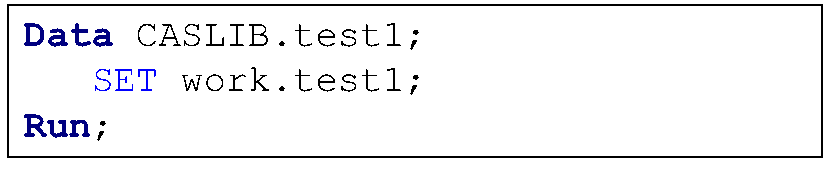Figure 9. DATA Step code to create distributed CAS table CASLIB.TEST1

To obtain the repeatable results, we need to control the sequence of rows within each BY group. We accomplish this by adding the new variable ROW_ID as the last variable to the BY statement in our DATA Step code, see figure 10.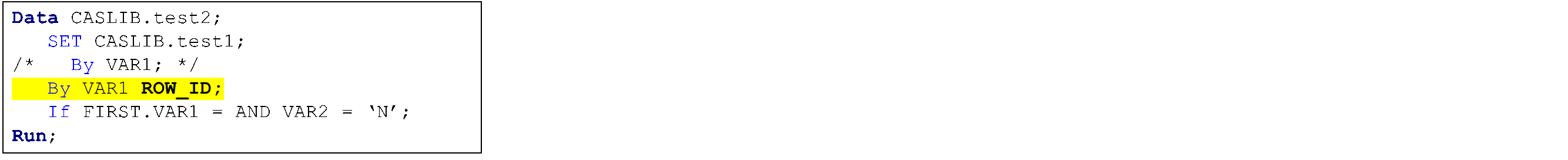Figure 10. Add ROW_ID as last variable of the BY group

Figure 11 shows us the output CAS table created by the code in figure 10. By adding the new variable ROW_ID and using that variable as the last variable of the BY statement, we are controlling the sequencing of rows within the BY groups for all 3 runs.

 VAR1 VAR2 ROW_ID 1 N 1

Figure 11. Distrusted CAS table CASLIB.TEST2

### Conclusion

With distributed DATA Step comes great opportunities to improve runtimes. It also means we need to understand differences between single-threaded processing of SAS7BDAT datasets that are stored on disk and distributed processing of CAS tables store in-memory. To help you with that journey I suggest you read the SAS Global Forum paper, Parallel Programming with the DATA Step: Next Steps.